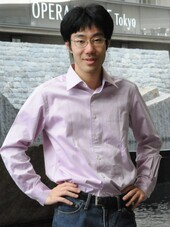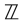# People

 Tomoyuki Abe PositionAssociate Professor (from 2016/07/01 ) show past_positionsResearch FieldMathematics Mathematics E-MailLast Update 2023/05/26

I am studying arithmetic geometry; especially, p-adic cohomology theory. Arithmetic geometry is a subject that tries to understand arithmetic equations, or more generally arithmetic varieties, by “geometric” methods. How, for example, can we get “topological information” of acoefficient equation? A naive answer would be, by considering the equation as a complex variety and taking the cohomology. Although we can get genus information with this method, we cannot see the difference between equations defined overand.

In the 60’s, Grothendieck defined étale cohomology on which arithmetic properties reflect. This important cohomology is an analog of singular cohomology. He also suggested more “analytic” cohomology, called crystalline cohomology, which is an analog of de Rham cohomology. I am studying a variation of crystalline cohomology called the arithmetic D-modules, and I am interested in the relationship between various cohomology theories from the viewpoint of Langlands program. Recently, some physicists pointed out links between the geometric Langlands program and “S-duality.” It is my dream to study arithmetics by using some insight from physics.

Back to Member List.